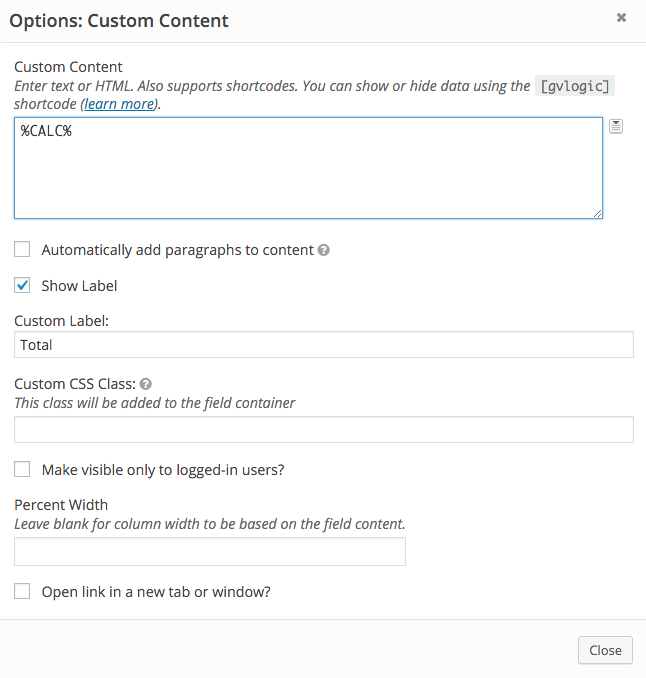# Making calculations using the custom content field

### Using the Math by GravityView plugin

Let's say you want to add the values from two fields: field "Number One" with an ID of 15 and "Number Two" with an ID of 37. You would just need to insert the Merge Tags of the fields and add them together inside the `[gv_math]` shortcode. Here's the code you would use:

```[gv_math scope="entry"] {Number One:15} + {Number Two:37} [/gv_math]
```

### Using custom code

Is it possible to sum up entries' fields and display the total number using a Custom Content field, without the Math by GravityView plugin? Yes, it is possible using the  gravityview/fields/custom/content_before hook!

In order to make sure you are addressing the correct custom content field, add a placeholder to the custom content field. For example, add `%CALC%`.Then, to enable this logic you would need to adapt and paste the following code into your theme's functions.php file (  see how ):